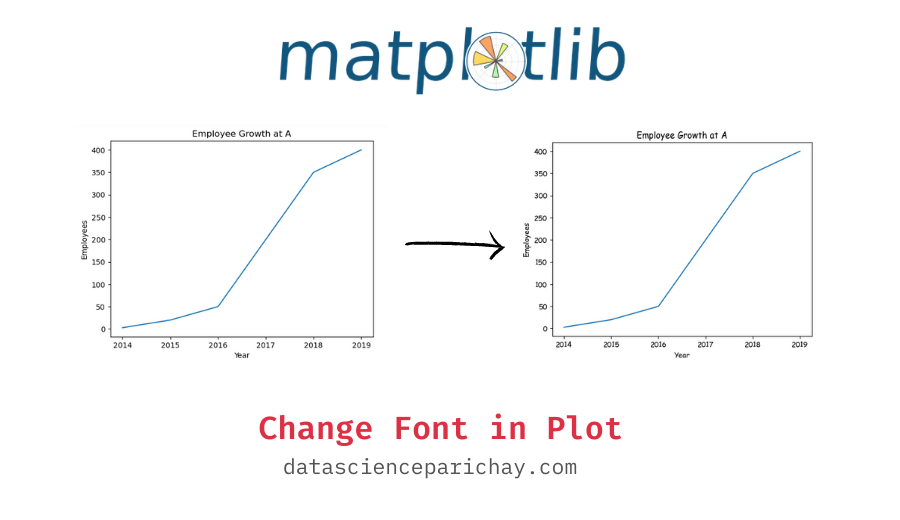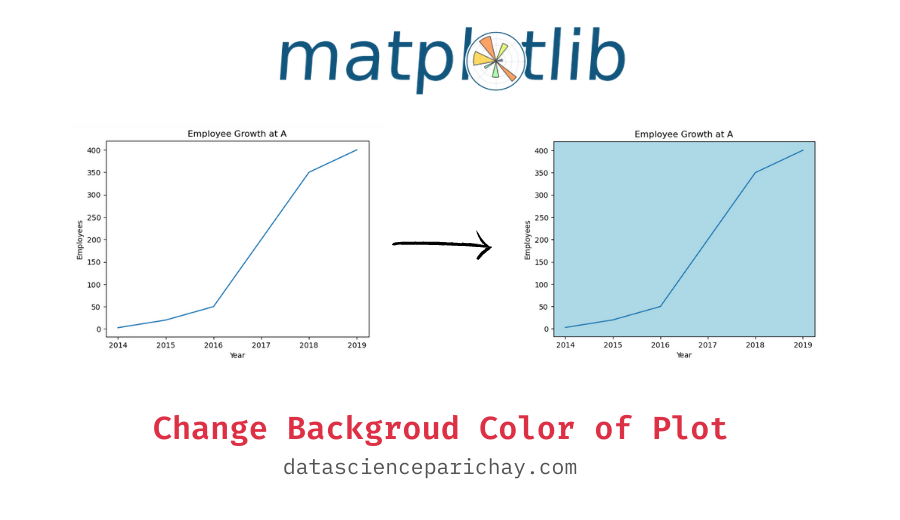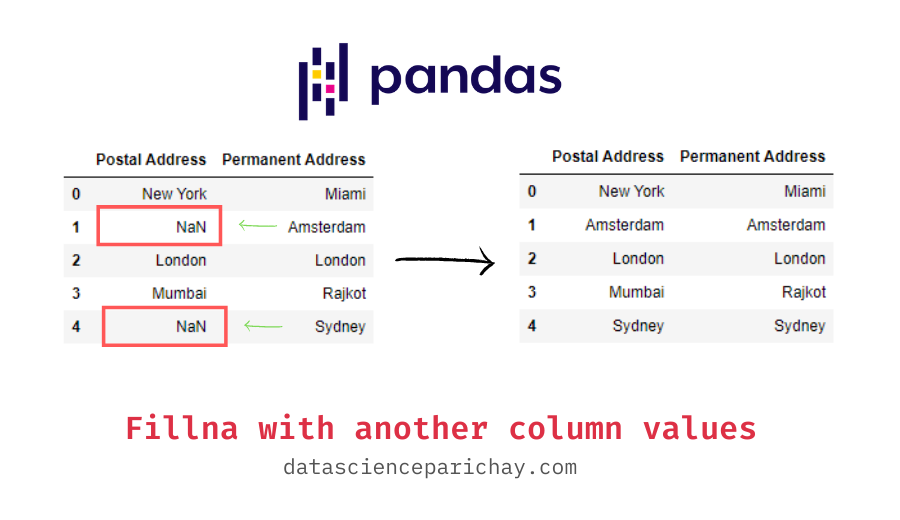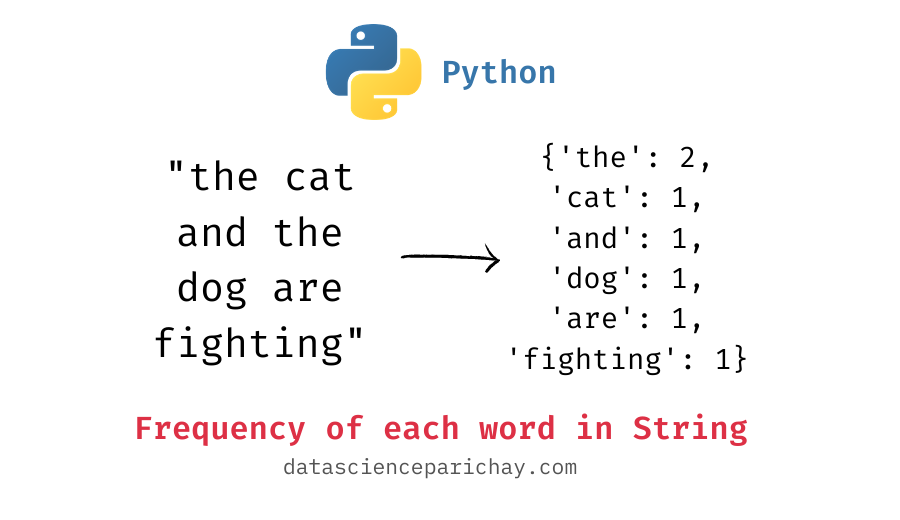## Change Font Type in Matplotlib plots

In this tutorial, we’ll look at how to change the font types of texts in matplotlib plots. How to change Font in Matplotlib? You can change the font globally for all the plots using rcParams. (See the syntax and examples below). You can also set the font individually for text components of a matplotlib axes …## Change Background Color of Plot in Matplotlib

In this tutorial, we’ll look at how to change the background color of plots in matplotlib. Note that, the background color here means the background color of the region bounded by the axes. Also referred to as the axes face color. How to change background color in matplotlib? To change the background color of matplotlib …## Change Font Size of elements in a Matplotlib plot

In this tutorial we’ll look at how to change the font size of text elements in a matplotlib plot. How to change the font size in matplotlib? You can change the global font size in matplotlib using rcparams. You can also change the font size of individual components such as tick labels, axes labels, axes …## Pandas – fillna with values from another column

In this tutorial, we’ll look at how to fill missing values (using fillna) in one column with values from another column of a pandas dataframe. Using fillna() to fill values from another column The pandas dataframe fillna() function is used to fill missing values in a dataframe. Generally, we use it to fill a constant …## Python – Frequency of each word in String

In this tutorial, we’ll look at how to count the frequency of each word in a string corpus in python. We’ll also compare the frequency with visualizations like bar charts. Count of each word in a string To count the frequency of each word in a string, you’ll first have to tokenize the string into …

Scroll to Top